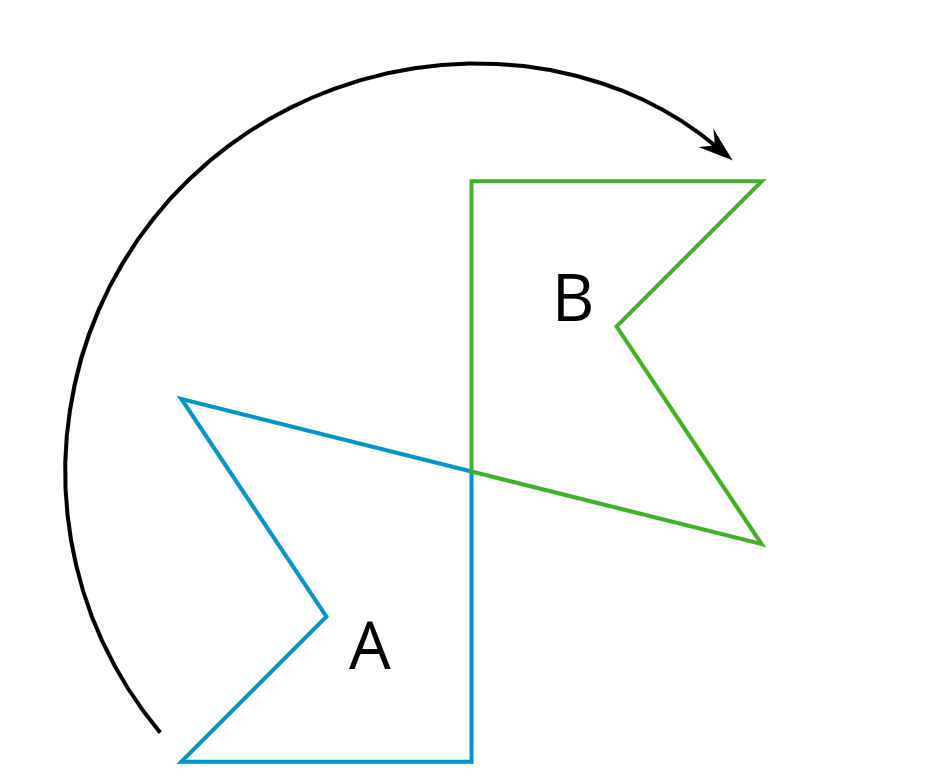# Lesson 6

Describing Transformations

### Lesson Narrative

Prior to this lesson, students have studied and classified different types of transformations (translations, rotations, reflections). They have practiced applying individual transformations and sequences of transformations to figures both on and off of a coordinate grid. In this lesson, they focus on communicating precisely the information needed to apply a sequence of transformations to a polygon on the coordinate grid. They must think carefully about what information they need (MP1) and request this information from their partner in a clear, precise way. They also explain why they need each piece of information (MP3). The coordinate grid plays a key role in this work, allowing students to communicate precisely about the locations of polygons and how they are transformed.

### Learning Goals

Teacher Facing

• Create a drawing on a coordinate grid of a transformed object using verbal descriptions.
• Identify what information is needed to transform a polygon. Ask questions to elicit that information.

### Student Facing

Let’s transform some polygons in the coordinate plane.

### Required Preparation

Print 1 copy of the blackline master for every 2 students. Cut them up ahead of time.

From the geometry toolkits, graph paper and tracing paper are especially helpful.

### Student Facing

• I can apply transformations to a polygon on a grid if I know the coordinates of its vertices.

### Glossary Entries

• clockwise

Clockwise means to turn in the same direction as the hands of a clock. The top turns to the right. This diagram shows Figure A turned clockwise to make Figure B.• coordinate plane

The coordinate plane is a system for telling where points are. For example. point $$R$$ is located at $$(3, 2)$$ on the coordinate plane, because it is three units to the right and two units up.

• counterclockwise

Counterclockwise means to turn opposite of the way the hands of a clock turn. The top turns to the left.

This diagram shows Figure A turned counterclockwise to make Figure B.

• image

An image is the result of translations, rotations, and reflections on an object. Every part of the original object moves in the same way to match up with a part of the image.

In this diagram, triangle $$ABC$$ has been translated up and to the right to make triangle $$DEF$$. Triangle $$DEF$$ is the image of the original triangle $$ABC$$.

• reflection

A reflection across a line moves every point on a figure to a point directly on the opposite side of the line. The new point is the same distance from the line as it was in the original figure.

This diagram shows a reflection of A over line $$\ell$$ that makes the mirror image B.

• rotation

A rotation moves every point on a figure around a center by a given angle in a specific direction.

This diagram shows Triangle A rotated around center $$O$$ by 55 degrees clockwise to get Triangle B.

• sequence of transformations

A sequence of transformations is a set of translations, rotations, reflections, and dilations on a figure. The transformations are performed in a given order.

This diagram shows a sequence of transformations to move Figure A to Figure C.

First, A is translated to the right to make B. Next, B is reflected across line $$\ell$$ to make C.

• transformation

A transformation is a translation, rotation, reflection, or dilation, or a combination of these.

• translation

A translation moves every point in a figure a given distance in a given direction.

This diagram shows a translation of Figure A to Figure B using the direction and distance given by the arrow.

The vertices in this polygon are labeled $$A$$, $$B$$, $$C$$, $$D$$, and $$E$$.